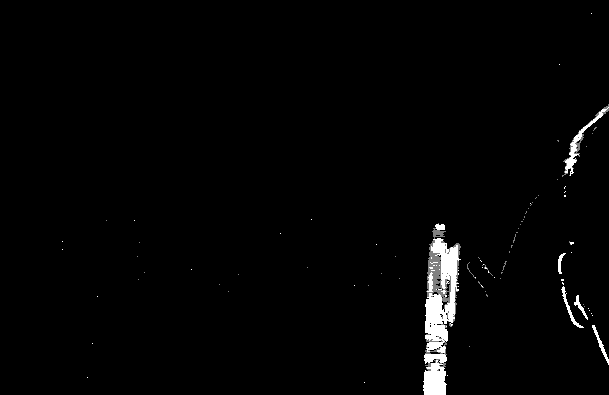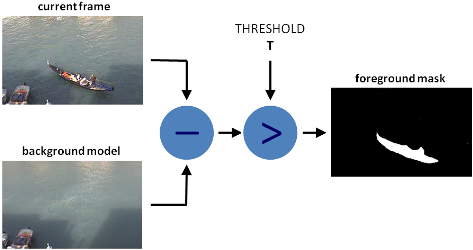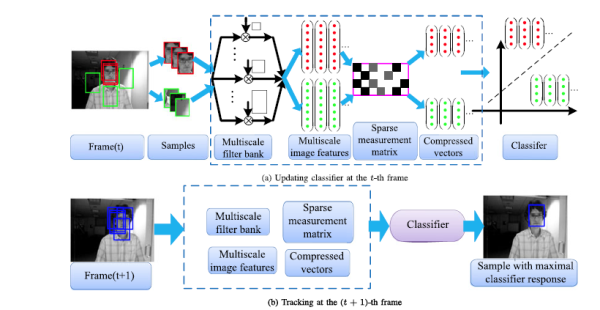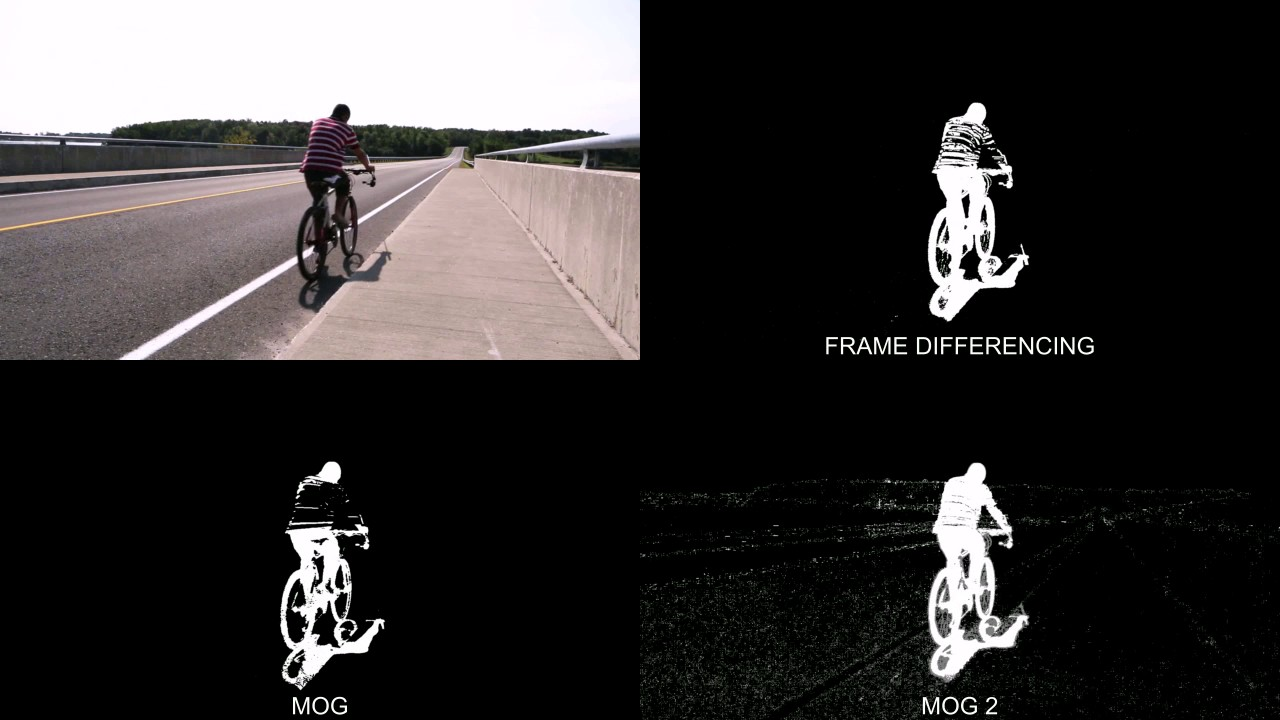#Object Tracking based on Deep Learning# What is Video tracking?

Target tracking is the process of locating moving targets in a video camera for a very wide range of real-world applications. Real-time target tracking is an important task for many computer vision applications, such as surveillance, perception-based user interfaces, augmented reality, object-based video compression and autonomous driving.Historically, there are many ways to track video targets: when you track all moving objects, the difference between the images becomes useful; for tracking the moving hand in the video, the average shift method based on skin color is the best solution; Model matching is a good technique for tracking an aspect of an object.

Since the results of the ImageNet 2012 challenge (opens new window), Deep Learning (and in particular, Convolutional Neural Networks (CNNs)) has become the main method for solving this kind of problem. Object tracking studies have therefore naturally integrated recognition models, which has made it possible to create tracking algorithms.

For more details, you can look at the following projects and tutorials related to the video track challenge:

Practical books that will allow you to learn the different aspects of video tracking:

# Conventional methods for object detection and tracking

1. Basic object detection

To make a baseline movement detection, given the difference between the "background" and the other frames, this method is still quite good, but you must first define the background frame, if it is outside, changes in lighting can cause a false detection. Therefore, this method is very limited. OpenCV offers a class, called BackgroundSubtractor, which is useful for splitting the foreground from the background. There are three background separators in OpenCV3: K-Nearest (KNN),* Gaussian Mixture (MOG2)*, and Geometric Multigid (GMG). The BackgroundSubtractor class used for video analysis, i.e. the BackgroundSubtractor class learns the context of each image. The BackgroundSubtractor class is often used to compare different frames as well as to record previous frames, which can be used to improve the results of motion analysis.2. Background splitter by MOG2

One of the basic features of the BackgroundSubtractor class is that it can compute shadows. This is absolutely essential for accurate playback of video images: by detecting shadows, it is possible to exclude shadow areas from the detected image (in threshold mode) so that real attributes can be focused.3. Background splitter by KNN

The images can be viewed from left to right: detected moving targets, background segmentation, thresholding after background segmentation.4. Kalman object tracking

Kalman is a Hungarian mathematician, who developed a filter from his PhD thesis work and the 1960 paper (opens new window) entitled "A New Approach to Linear Filtering and Prediction Problems".

Kalman filtering has been applied in many domains, particularly in the navigation guidance of aircraft and missiles. The Kalman filter works repeatedly on a noisy input data stream (such as a video input in computer vision) and produces a statistically optimal estimate of the state of the underlying system (such as the position in the video).

The Kalman filter algorithm is divided into two phases:

• Prediction phase: the Kalman filter uses the covariance computed from the current position to estimate the target's new position.
• Update phase: the Kalman filter stores the target position and calculates the corrected covariance for the next iteration.# Deep Learning based methods for object detection and tracking

In recent years, Deep Learning methods have been successfully applied in the field of object tracking and are gradually exceeding traditional performance methods. In this section, we will present current target tracking algorithms based on Deep Learning.

1. Trends in object tracking

Unlike the trend towards of Deep Learning in the visual domain, such as detection and recognition, the application of this paradigm in the object tracking domain is not seamless. The main problem is the lack of learning data: one of the complications of the deep model comes from the effective learning of a large number of labelled learning data, while target tracking only provides the context for selecting the first image as learning data. In this case, it is difficult to train a deep model from scratch at the beginning of the tracking. Currently, the target tracking algorithm based on Deep Learning takes several ideas to solve this problem, including the following and finally the recurrent neural network in the current tracking field to solve the target tracking problem. Where training data for target tracking is very limited, complementary non-supervised training data is used for pre-training to achieve a high-level feature epresentation of object, and in actual tracking, using the limited sample information from the current tracking target. The accuracy of the pre-training model confers a higher classification performance on the model for the current tracking goal, which significantly reduces the need to track target training samples and improves the performance of the tracking algorithm.

2. Overall multi-target tracking process

In order to track a target, first this target is detected. This step is called target detection, then the target in each image is mapped based on the detection result. Today, there are multi-target detectors, such as SSD (opens new window) and YOLO (opens new window), etc.

3. State-of-the-art methods for object tracking

# 3.1.GOTURN

A further great strength of deep learning is the end-to-end learning process. We believe that this opens up a promising future for tracking. Here is an example of the GOTURN method (opens new window). GOTURN's current method has been included in OpenCV 3.2.0 development version.GOTURN involves a convolution network based on the input of a pair of images using the ALOV300+ video sequence set and the ImageNet sensing data set, and generates the position change from the previous frame in the detection area to obtain the target's position on the current frame.

# 3.2. Specific-target tracking

In practice, a significant aspect of tracking is the tracking of specific objects, such as face tracking, gesture tracking and human tracking. Tracking a particular object is different from the approach described above and relies more on the training of a particular detector. Due to its obvious features, face tracking is mainly implemented through detection task, such as the state-of-the-art Viola-Jones detection model and the current face detection or face point detection model using Deep learning.

# 3.3. Compression tracking

This approach involves using the compressed detection method to represent feature maps, by achieving dimensional reduction and obtaining small-size cues in order to capture large-size feature space (The block diagram is shown in Figure).# Use case: Motion Tracker

Here, we will see how to track the motion of moving objects in the video using OpenCV 3.0 and basic techniques (MOG2). Motion tracking is used to track the motion of objects and then transmit the detected information to an application for further processing.Basic dependencies:

• OpenCV 3.0;
• Numpy lib.

The tracker implementation is as follows:

%%writefile points.py
import numpy as np
import numpy.linalg.linalg as la
import matplotlib.pyplot as plt

fst = lambda x: x
snd = lambda x: x

distance = lambda p1,p2: la.norm(p1 - p2)

matchPaths = lambda r, a, paths: [neighborhoodPath(r,paths,ai) for ai in a]

neighborhoodPath = lambda r, paths, pnt: [path for path in paths if distance(path,pnt) < r]

def itemMatcher(choice, items):
items = list(sorted(items, key = lambda x: len(x)))
accumulator = []
for index in range(len(items)):
(a, bs) = items[index]
if bs == []:
accumulator.append((a, None))
else:
b = choice(a,bs)
accumulator.append((a, b))
for ind in range(len(items)):
(a2, bs2) = items[ind]
if any(np.array_equal(b,belement) for belement in bs):
bs2 = [belement for belement in bs2 if not np.array_equal(belement, b)]
items[ind] = (a2, bs2)
return accumulator

def extendPaths(r, paths, scatter, filterWith, noisy = False, discard = True):
matches = matchPaths(r,scatter,paths)
zipped = zip(scatter, matches)
def choice(point, pathOptions):
return max(pathOptions,key=len)
def combine(tup):
(pnt, val) = tup
if val == None:
if not noisy:
return [pnt]
else:
return []
else:
return [pnt] + val
lst = itemMatcher(choice, zipped)
extended_paths = map(snd,lst)
return filter(lambda x: x != [], map(combine,lst))
unextended_paths = [p for p in paths if not array_in(p,extended_paths)]
unextended_paths = filter(filterWith, unextended_paths)
return ( unextended_paths, filter(lambda x: x!=[], map(combine, lst)) ) # First element is paths to be archived, second element is the extended paths

def array_in(arr, lst):
return any(np.array_equal(arr,elem) for elem in lst)

import pickle

def stringPaths(r, scatters):
paths = []
for sc in scatters:
paths = extendPaths(r, paths, sc)
return paths

def plotit(paths):
p = [reduce(lambda x,y: np.append(x,y,axis=0),pa) for pa in paths]
p = map(lambda x: x.T, p)
plt.hold(True)
map(lambda x: plt.plot(x,x,'x'),p)

def shortcut(r, filename):
def rawpoints(filename):
plotit(scatters)
from __future__ import division
import numpy as np
import cv2
import scipy
import pickle
import points

from sys import argv

OBJ = True
### THE SETTING PARAMETERS FOR VIDEO.MP4
if OBJ:
KERN_SIZE = 8
THRESHOLD_AT = 100
INPUT_SIZE_THRESHOLD = 75
MIN_PATH_SIZE = 10
WRITE_TO_FILE = True
###END PARAMETERS
else:
KERN_SIZE = 8
THRESHOLD_AT = 127
INPUT_SIZE_THRESHOLD = 150
MIN_PATH_SIZE = 10
WRITE_TO_FILE = True

video_output = "output_tracking.avi"

def avgit(y):
return x.sum(axis=0)/np.shape(y)
def plotp(p,mat,color=0):
mat[p[0,1],p[0,0]] = color

if len(argv) != 3:
cap = cv2.VideoCapture('vid1.mp4')
else:
cap = cv2.VideoCapture(argv)
video_outputfile = argv
fourcc = cv2.VideoWriter_fourcc(*'XVID')
height, width, layers = frame.shape
video_out = cv2.VideoWriter(video_outputfile, fourcc, 30, (width, height), True)
print video_out.isOpened()

fgbg = cv2.createBackgroundSubtractorMOG2()
bwsub= cv2.createBackgroundSubtractorMOG2()

kernlen = KERN_SIZE
kern = np.ones((kernlen,kernlen))/(kernlen**2)
ddepth = -1
def blur(image):
return cv2.filter2D(image,ddepth,kern)
def blr_thr(image, val=127):
return cv2.threshold(blur(image),val,255,cv2.THRESH_BINARY)
def normalize(image):
s = np.sum(image)
if s == 0:
return image
return height*width* image / s
#collection = []
paths = []
archive = []

thresh_at = THRESHOLD_AT
THIS_MUCH_IS_NOISE = INPUT_SIZE_THRESHOLD

while(cap.isOpened()):
gray = cv2.cvtColor(frame, cv2.COLOR_BGR2GRAY)

cons = res
scatter = map(avgit, cons)
filterWith = lambda x: len(x) > MIN_PATH_SIZE and np.std(x) > MINIMUM_PATH_STD
(toArchive, paths) = points.extendPaths(r, paths, scatter, filterWith, noisy=(len(scatter) > THIS_MUCH_IS_NOISE), discard=False)
archive += toArchive
for path in archive:
#color = 255
cv2.polylines(img, np.int32([reduce(lambda x,y: np.append(x,y,axis=0), path)]), 0, (255,0,0))
for path in paths:
cv2.polylines(img, np.int32([reduce(lambda x,y: np.append(x,y,axis=0), path)]), 1, (0,0,255))
cv2.imshow('frame', img)
video_out.write(img)
if cv2.waitKey(1) & 0xFF == ord('q'):
break
cap.release()
video_out.release()
cv2.destroyAllWindows()

if WRITE_TO_FILE:
pickle.dump(archive, open('my_path' + str(np.floor(1000*np.random.rand())) + '.pickle','w'))